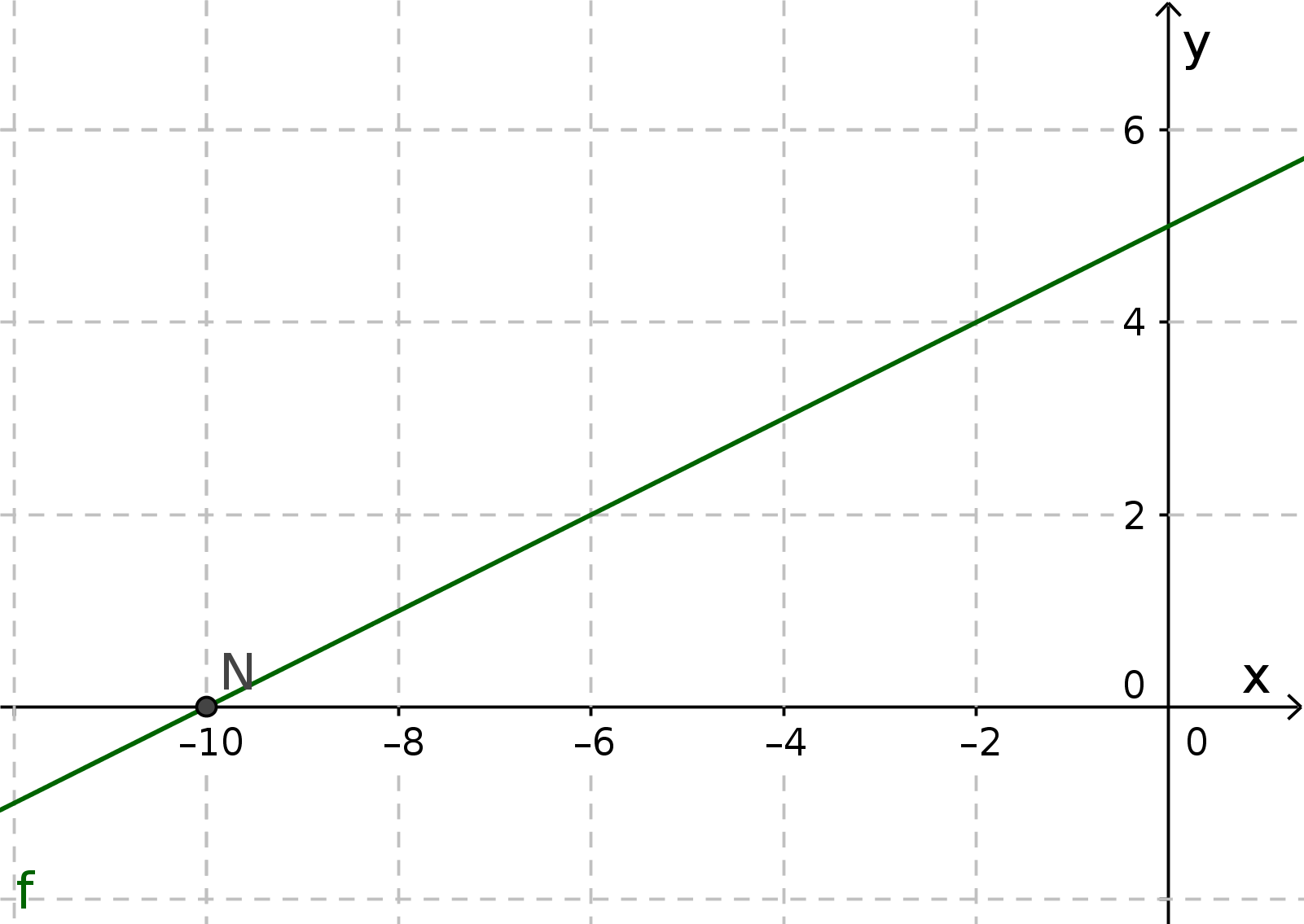Math Linear functions Calculating Zeros

# Calculating Zeros

The x-coordinate of the intersection of a graph with the x-axis is called the Zero.
A zero is thus present exactly when the y-coordinate is zero.

i

### Method

1. Set function equal to zero ($x_{ N } \Leftrightarrow f(x_N)=0$ and $mx+n=0$)
2. Solve equation for $x$

### Example

Calculate the zero of the function with the function equation: $f(x)=0.5x+5$1. #### Set function equal to zero

$f(x)=0$
$0.5x+5=0$
2. #### Solve equation for $x$

$0.5x+5=0\quad|-5$
$0.5x=-5\quad|:0.5$
$x_{ N }=-10$